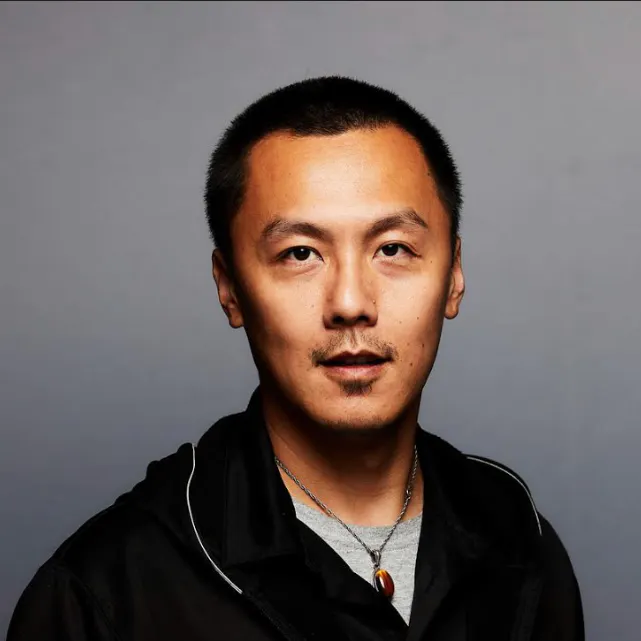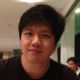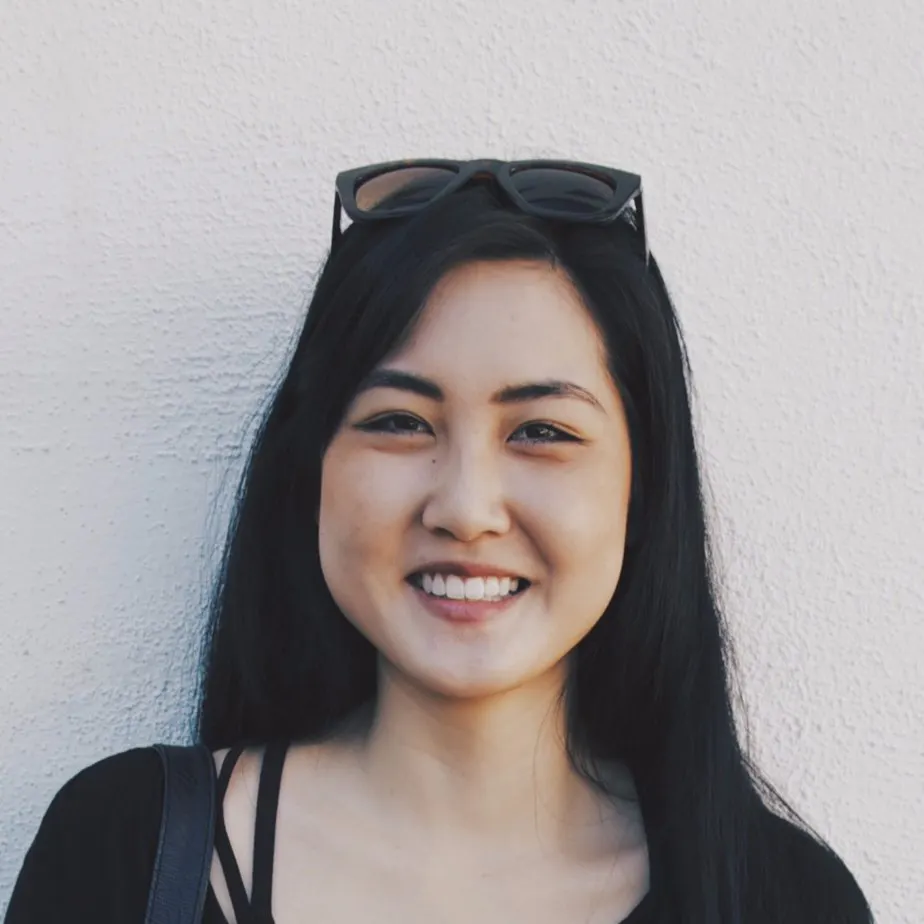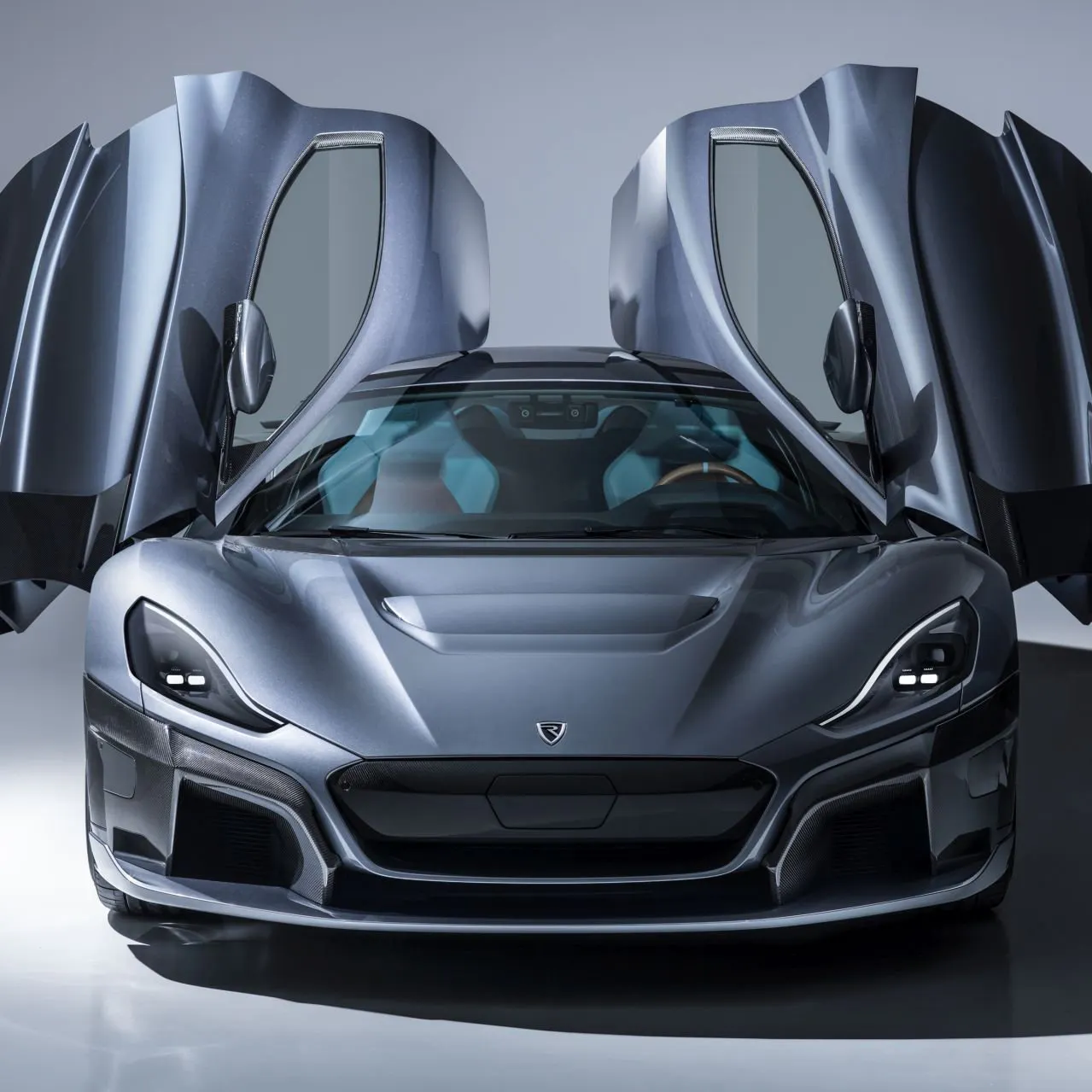Published © GPL3+

# MixPose

AI on the Edge streaming platform for yoga instructors and fitness coaches.

AdvancedFull instructions providedOver 1 day6,061

## Things used in this project

### Hardware components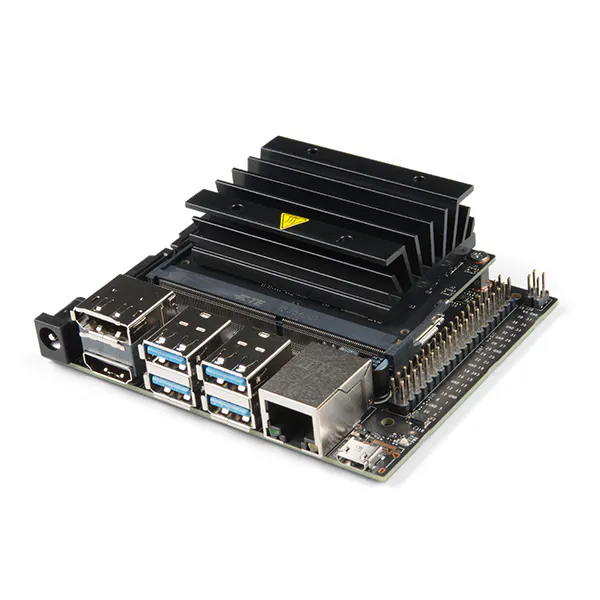NVIDIA Jetson Nano Developer Kit
×1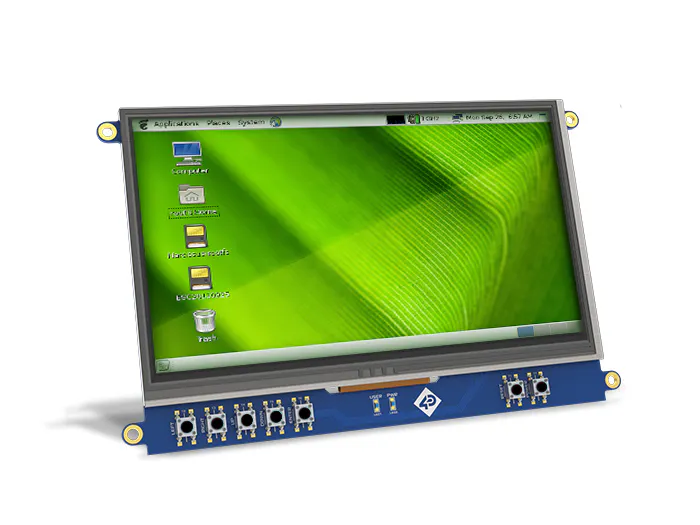Seeed 7 Inch LCD Cape for Beagle Bone Black - Touch Display
×1
 NVIDIA Shield TV Pro
×1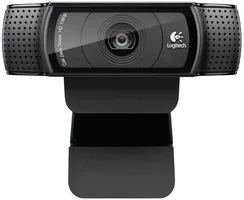Webcam, Logitech® HD Pro
×1

### Software apps and online services

 NVIDIA JetPack SDK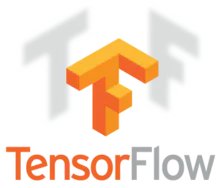TensorFlow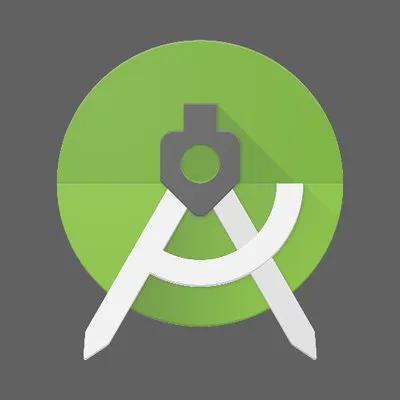Android Studio

### Hand tools and fabrication machines3D Printer (generic)

Read more

## Schematics

### Jetson Schematic

Jetson Schematic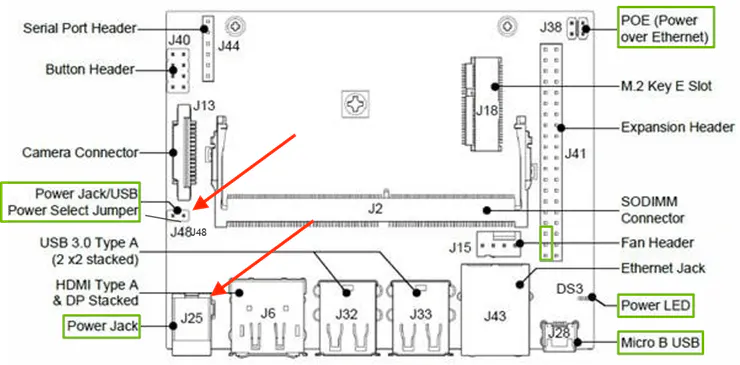## Code

### mixpose.py

Python
Code to run MixPose
```# Import packages
import os
import cv2
import numpy as np
import tensorflow as tf
import sys
import time
import argparse
import posenet

parser = argparse.ArgumentParser()
parser.add_argument('--model', type=int, default=101)
parser.add_argument('--cam_id', type=int, default=0)
parser.add_argument('--cam_width', type=int, default=640)
parser.add_argument('--cam_height', type=int, default=480)
parser.add_argument('--scale_factor', type=float, default=0.7125)
parser.add_argument('--file', type=str, default=None, help="Optionally use a video file instead of a live camera")
args = parser.parse_args()

# This is needed since the notebook is stored in the object_detection folder.
sys.path.append("..")

# Import utilites
from utils import label_map_util
from utils import visualization_utils as vis_util

# Name of the directory containing the object detection module we're using
MODEL_NAME = 'inference_graph'

# Grab path to current working directory
CWD_PATH = os.getcwd()

# Path to frozen detection graph .pb file, which contains the model that is used
# for object detection.
PATH_TO_CKPT = os.path.join(CWD_PATH,MODEL_NAME,'frozen_inference_graph.pb')

# Path to label map file
PATH_TO_LABELS = os.path.join(CWD_PATH,'training','labelmap.pbtxt')

# Number of classes the object detector can identify
NUM_CLASSES = 6

## Load the label map.
# Label maps map indices to category names, so that when our convolution
# network predicts `5`, we know that this corresponds to `king`.
# Here we use internal utility functions, but anything that returns a
# dictionary mapping integers to appropriate string labels would be fine
label_map = label_map_util.load_labelmap(PATH_TO_LABELS)
categories = label_map_util.convert_label_map_to_categories(label_map, max_num_classes=NUM_CLASSES, use_display_name=True)
category_index = label_map_util.create_category_index(categories)

# Load the Tensorflow model into memory.
detection_graph = tf.Graph()
with detection_graph.as_default():
od_graph_def = tf.GraphDef()
with tf.gfile.GFile(PATH_TO_CKPT, 'rb') as fid:
serialized_graph = fid.read()
od_graph_def.ParseFromString(serialized_graph)
tf.import_graph_def(od_graph_def, name='')

sess = tf.Session(graph=detection_graph)
model_cfg, model_outputs = posenet.load_model(args.model, sess)
output_stride = model_cfg['output_stride']
start = time.time()
frame_count = 0
# Define input and output tensors (i.e. data) for the object detection classifier

# Input tensor is the image
image_tensor = detection_graph.get_tensor_by_name('image_tensor:0')

# Output tensors are the detection boxes, scores, and classes
# Each box represents a part of the image where a particular object was detected
detection_boxes = detection_graph.get_tensor_by_name('detection_boxes:0')

# Each score represents level of confidence for each of the objects.
# The score is shown on the result image, together with the class label.
detection_scores = detection_graph.get_tensor_by_name('detection_scores:0')
detection_classes = detection_graph.get_tensor_by_name('detection_classes:0')

# Number of objects detected
num_detections = detection_graph.get_tensor_by_name('num_detections:0')

# Initialize webcam feed
video = cv2.VideoCapture(0)
ret = video.set(3,640)
ret = video.set(4,480)

while(True):

input_image, display_image, output_scale = posenet.read_cap(
video, scale_factor=args.scale_factor, output_stride=output_stride)

heatmaps_result, offsets_result, displacement_fwd_result, displacement_bwd_result = sess.run(
model_outputs,
feed_dict={'image:0': input_image}
)

pose_scores, keypoint_scores, keypoint_coords = posenet.decode_multi.decode_multiple_poses(
heatmaps_result.squeeze(axis=0),
offsets_result.squeeze(axis=0),
displacement_fwd_result.squeeze(axis=0),
displacement_bwd_result.squeeze(axis=0),
output_stride=output_stride,
max_pose_detections=10,
min_pose_score=0.15)

keypoint_coords *= output_scale

# TODO this isn't particularly fast, use GL for drawing and display someday...
skeleton_frame = posenet.draw_skel_and_kp_figureonly(
display_image, pose_scores, keypoint_scores, keypoint_coords,
min_pose_score=0.15, min_part_score=0.1)

#cv2.imshow('posenet', overlay_image)

# Acquire frame and expand frame dimensions to have shape: [1, None, None, 3]
# i.e. a single-column array, where each item in the column has the pixel RGB value
#ret, frame = video.read()
frame_expanded = np.expand_dims(skeleton_frame, axis=0)

# Perform the actual detection by running the model with the image as input
(boxes, scores, classes, num) = sess.run(
[detection_boxes, detection_scores, detection_classes, num_detections],
feed_dict={image_tensor: frame_expanded})

# Draw the results of the detection (aka 'visulaize the results')
vis_util.visualize_boxes_and_labels_on_image_array(
skeleton_frame,
np.squeeze(boxes),
np.squeeze(classes).astype(np.int32),
np.squeeze(scores),
category_index,
use_normalized_coordinates=True,
line_thickness=6,
min_score_thresh=0.75)

combined = cv2.add(display_image, skeleton_frame)

# All the results have been drawn on the frame, so it's time to display it.
cv2.imshow('Skeleton Tracker', combined)

frame_count += 1
# Press 'q' to quit
if cv2.waitKey(1) == ord('q'):
break
print('Average FPS: ', frame_count / (time.time() - start))

# Clean up
video.release()
cv2.destroyAllWindows()
```

### MixPose Jetson Repo

MixPose Jetson Repo

## Credits

### Peter Ma

49 projects • 356 followers
Prototype Hacker, Hackathon Goer, World Traveler, Ecological balancer, integrationist, technologist, futurist.

### Ethan Fan

9 projects • 29 followers
Mobile, Watch, and Glass Developer

### Sarah Han

13 projects • 66 followers
Software Engineer, Design, 3D

### Natka Wojcik

1 project • 2 followers

### Serena Xu

1 project • 0 followers
Product Designer, Frontend Developer
Thanks to Bensound.